# Spatial Sampling and Interpolation

Many phenomena that we want to understand occur over wide geographical areas. While there are numerous cases where remote sensing techniques using satellite or aerial photography can be used to capture such data, there are often situations where it is not possible to directly capture a complete surface data set.

In such cases, you will need take sample measurements at selected points and then interpolate the values for the areas in between.

For example, it would be impossible to completely measure the amount and quality of gold ore deep under a large field. But if we could take samples from handful of drilled boreholes and estimate the nature of the deposit in the areas between those boreholes, that would allow us to estimate the volume and quality of the reserve, and know whether further investment would be justified.

While the techniques for spatial interpolation are often domain-specific and, accordingly, complex, there are some fundamental spatial sampling and interpolation techniques that are applicable across a wide variety of domains, and this tutorial will introduce how to execute those techniques in R.

## Surfaces and Rasters

The values of a variable across space are commonly conceived of as surfaces. While the imagery of a surface implies elevation (height), variables modeled with surfaces can be anything that has a distribution across space, such as prevalance of an animal species, temperature, or concentrations of pollutants.

Surfaces are commonly represented as raster grids of evenly spaced cells. In R, the raster library provides a wide variety of functions for building, manipulating, and analysing surfaces represented with rasters.

For example, digital elevation models (DEM) are rasters of elevation data. The US Geological Survey distributes DEMs via The National Map. The example below uses this DEM data for an area around Spokane, WA. The plot() function gives a false-color view of the data.

```dem = raster("imgn48w118_1.img")
plot(dem)
```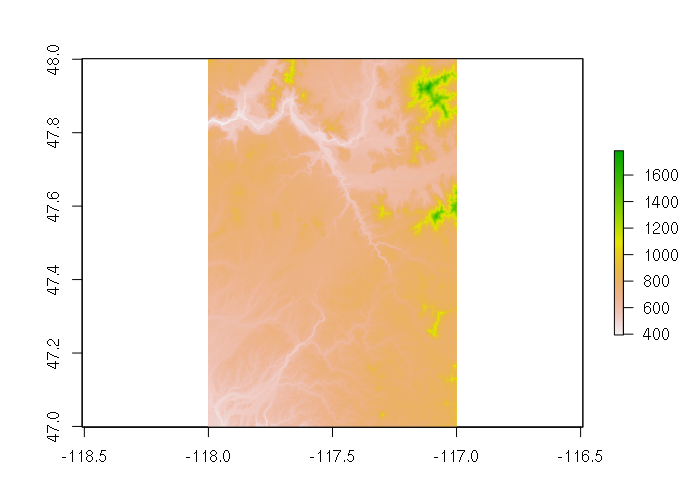Eastern Washington Digital Elevation Model False-Color View (USGS)

The persp() function creates a perspective visualization of surface data.

The exact parameters needed to make the visualization useful will depend on your data. In the example below:

• border=NA turns the borders off, because with high-resolution data like this, borders turn the surface black.
• shade=0.5 enables hillshading, giving the effect of light and shadows
• scale=F preserves the aspect ratio of cells (rather than expanding them to fit the display) and expand=0.0001 reduces the scaling of elevation since x/y coordinates are in degrees but elevation is in meters
```persp(dem, col='tan', scale=F, expand = 0.0001, shade=0.5, border=NA)
```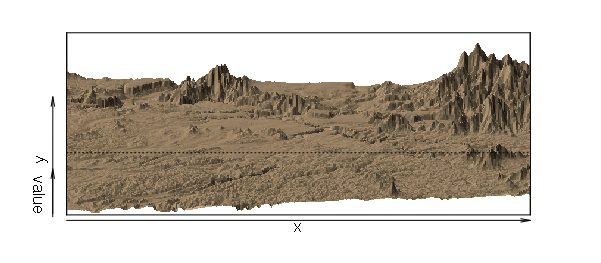Eastern Washington Digital Elevation Model Perspective View (USGS)

## Spatial Sampling

There are a variety of spatial probability sampling approaches that are similar to their non-spatial counterparts. de Smith, Goodchild, and Longley (2015) point out four systematic approaches:

• Regularly spaced X/Y locations on a grid
• Random X/Y locations
• Regular grid with random X/Y offsets
• Regular grid with random row or column offsets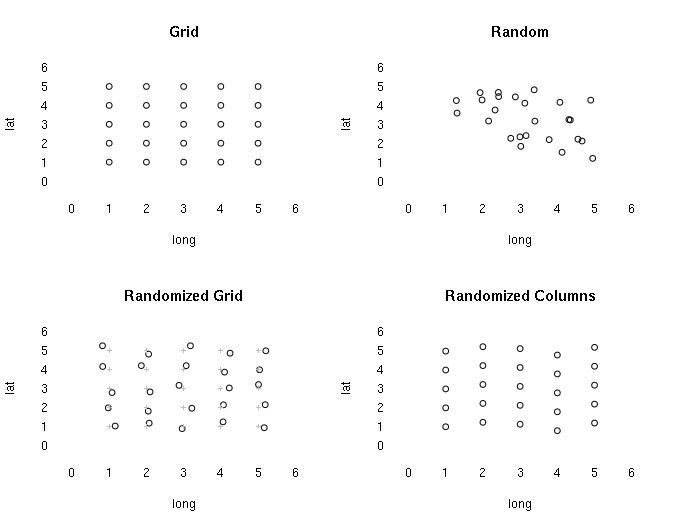Types of Spatial Sampling Grids

However, as with other types of sampling like polls and surveys, strict adherence to rigorous regularity or randomness may not be practical. Nonprobability sampling techniques like convenience sampling where samples are gathered at the most accessible or permissible locations may be necessary.

Although the statistical validity of inferences made from that data is reduced and uncertainty is increased, depending on the nature of the sampled phenomenon and the desired level of rigor, such data may be of value for preliminary research or conclusions that are presented with caveats.

## Example GPX Data

The following examples will demonstrate spatial interpolation using GPX data gathered as a convenience sample by traversing walkways on the Eastern Washington University campus. This demonstration GPX file can be downloaded HERE....

While spatial interpolation is used for a wide variety of applications other than elevation, elevation is a readily-understandable and accessible example for demonstration.

GPX is an XML-based format used to store GPS tracking information. There are a wide variety of apps available for both iPhone and Android devices that can create GPX files using the GPS receivers in all contemporary smartphones.

GPX files can contain a number of different sets of points that are treated as layers by OGR, although some layers may be empty. You can list the layers with the ogrListLayer() function from the rgdal library. You can list information about a layer with the ogrInfo() function.

```> library(rgdal)

> ogrListLayers("2017-05-010-175527.gpx")
 "waypoints"    "routes"       "tracks"       "route_points" "track_points"
attr(,"driver")
 "GPX"
attr(,"nlayers")
 5

> ogrInfo("2017-05-010-175527.gpx", "track_points")
Source: "2017-05-010-175527.gpx", layer: "track_points"
Driver: GPX; number of rows: 261
Feature type: wkbPoint with 2 dimensions
Extent: (-117.5863 47.48662) - (-117.5771 47.49273)
CRS: +proj=longlat +datum=WGS84 +no_defs
Number of fields: 26
name type length typeName
1           track_fid    0      0  Integer
2        track_seg_id    0      0  Integer
3  track_seg_point_id    0      0  Integer
4                 ele    2      0     Real
5                time   11      0 DateTime
6              magvar    2      0     Real
7         geoidheight    2      0     Real
8                name    4      0   String
9                 cmt    4      0   String
10               desc    4      0   String
11                src    4      0   String
12         link1_href    4      0   String
13         link1_text    4      0   String
14         link1_type    4      0   String
15         link2_href    4      0   String
16         link2_text    4      0   String
17         link2_type    4      0   String
18                sym    4      0   String
19               type    4      0   String
20                fix    4      0   String
21                sat    0      0  Integer
22               hdop    2      0     Real
23               vdop    2      0     Real
24               pdop    2      0     Real
25      ageofdgpsdata    2      0     Real
26             dgpsid    0      0  Integer
```

The readOGR() function will read points from a GPX file into a SpatialPointsDataFrame object:

```> points = readOGR(dsn='2017-05-010-175527.gpx', 'track_points', stringsAsFactors=F)
OGR data source with driver: GPX
Source: "2017-05-010-175527.gpx", layer: "track_points"
with 261 features
It has 26 fields
> names(points)
 "track_fid"          "track_seg_id"       "track_seg_point_id"
 "ele"                "time"               "course"
 "speed"              "magvar"             "geoidheight"
 "name"               "cmt"                "desc"
 "src"                "url"                "urlname"
 "sym"                "type"               "fix"
 "sat"                "hdop"               "vdop"
 "pdop"               "ageofdgpsdata"      "dgpsid"
> plot(points)
```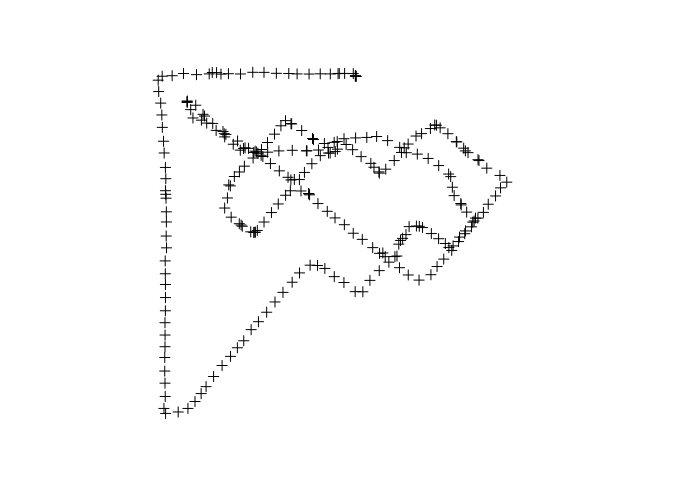Raw Point Map of Example GPS Points

If your data includes points gathered outside the particular area of interest, they can be removed by subsetting as with any other SpatialPointsDataFrame. For example, the following selection removes possibly errant points from the beginning and ending of the survey.

```> range(points\$time)
 "2017/05/01 23:52:11+00" "2017/05/02 00:55:30+00"
> points = points[(points\$time >= '2017/05/01 23:54') & (points\$time < '2017/05/02 00:50:00'),]
> range(points\$time)
 "2017/05/01 23:54:04+00" "2017/05/02 00:49:44+00"
> spplot(points, zcol='ele')
```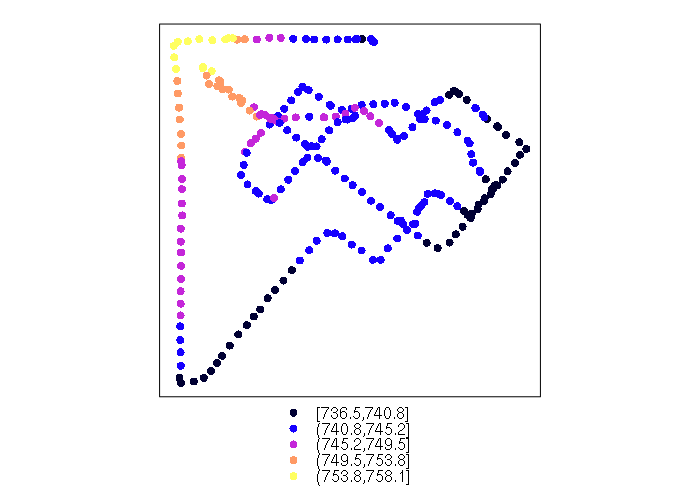Subset Point Map of Example GPS Points

An OpenStreetMap base map can be used to give geographic context:

```library(OpenStreetMap)
basemap = openmap(upperLeft = c(47.494, -117.588), lowerRight = c(47.486, -117.576), type="osm")

palette = colorRampPalette(c("#a6611a", "#018571"))
breaks = qunif(seq(0, 1, 0.2), min=min(points\$ele), max = max(points\$ele))
colors = palette(5)[cut(points\$ele, breaks)]

plot(basemap)
plotpoints = spTransform(points, osm())
points(plotpoints, pch=19, col=colors)
```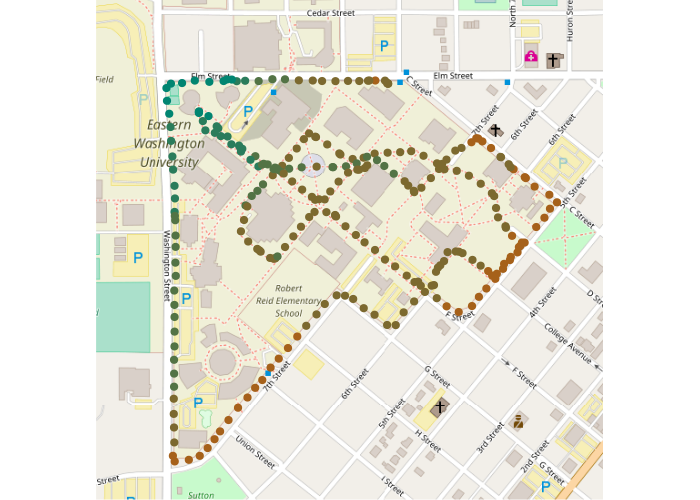Points Over an OSM Base Map

Spatial interpolation will be more meaningful if a planar coordinate system is used, where coordinates represent distances on the ground. The State Plane Coordinate system is commonly used in the US. Note that the transformation casuses the map to be tilted off the north-south axis due to the conic transformation needed to make ground distances accurate.

```# NAD 1983 HARN StatePlane Washington North FIPS 4601
# http://spatialreference.org/ref/esri/102348/

washington_north = CRS("+proj=lcc +lat_1=47.5 +lat_2=48.73333333333333
+lat_0=47 +lon_0=-120.8333333333333 +x_0=500000 +y_0=0 +ellps=GRS80 +units=m +no_defs")

points = spTransform(points, washington_north)
basemap = openproj(basemap, washington_north)

plot(basemap)
points(points, pch=19, col=colors)
```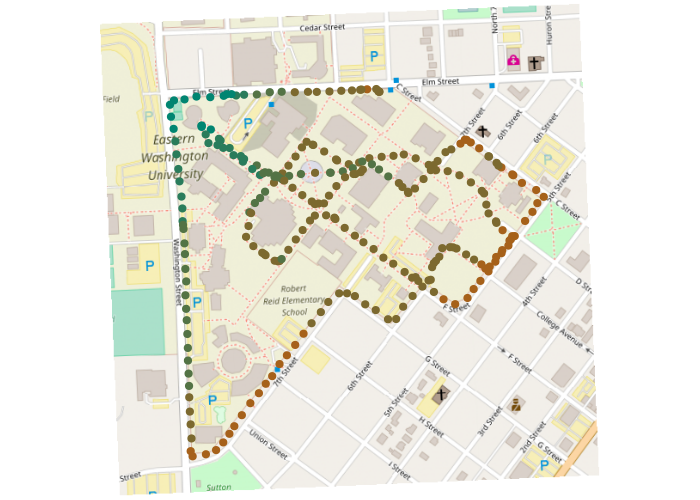Points and Basemap Reprojected to State Plane Coordinates

## Rasterization

The first step to creating a surface interpolated from points is to define the spatial extent and cell dimension for the raster used to represent the surface.

The function below creates a template raster with no values that can be used by subsequent functions to create rasters with the same dimensions. Because the state plane coordinate system used is in feet, the pixel size of 25 means each pixel is 25 feet square. The value you use will be based on how much spatial resolution you need. Higher resolutions require more memory, and imply higher spatial accuracy, which may not be justified if you do not have enough data.

```library(raster)

pixelsize = 25
box = round(extent(points) / pixelsize) * pixelsize
template = raster(box, crs = washington_north,
nrows = (box@ymax - box@ymin) / pixelsize,
ncols = (box@xmax - box@xmin) / pixelsize)
```

This template raster has no data, but the rasterize() function from the raster library can be used to visualize the sampled data in the context of the surface that will be created, and assess whether the pixel size is appropriate for your data. The fun=mean parameter is passed to use the mean() function to find the average elevation when multiple points overlap single raster pixel.

```points\$PRESENT = 1
pointraster = rasterize(points, template, field = 'ele', fun = mean)
plot(pointraster)
```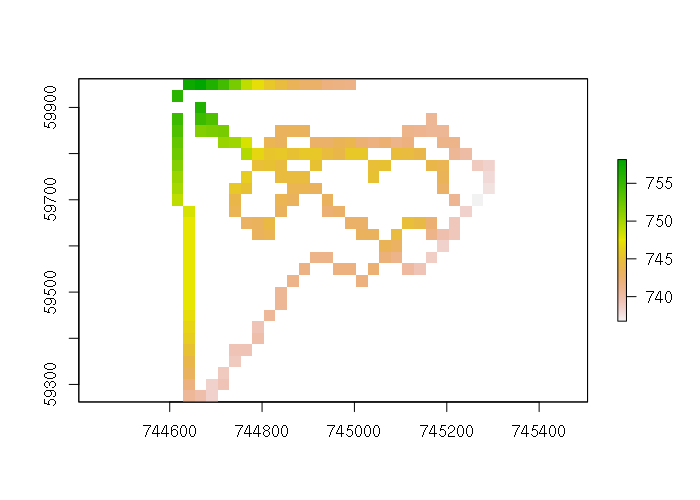Rasterized Sample Points

## Thin Plate Spline

Interpolation techniques are based on models that use patterns in the data (and sometimes other fields) to predict what missing values should be. There are a variety of different models that can be fed into the interpolate() function from the raster library, along with a template raster, to create interpolated surfaces.

A simple model is a thin plate spline, which can be created using the Tps() function from the fields library. Thin plate splines were introduced to geometric design Jean Duchon in 1977.

As the name implies, a thin plate spline can be visualized metaphorically as the shape resulting from draping a slightly rigid sheet of metal over a set of elevation points. This creates a smooth surface.

Because this is a geometric technique, it may not fully model the processes causing differentiation in your data. While you may get a surface that looks attractive, it may not accurately represent the actual modeled phenomenon.

```library(fields)
spline = Tps(points@coords, points\$ele)
splined = interpolate(template, spline)
plot(splined)
```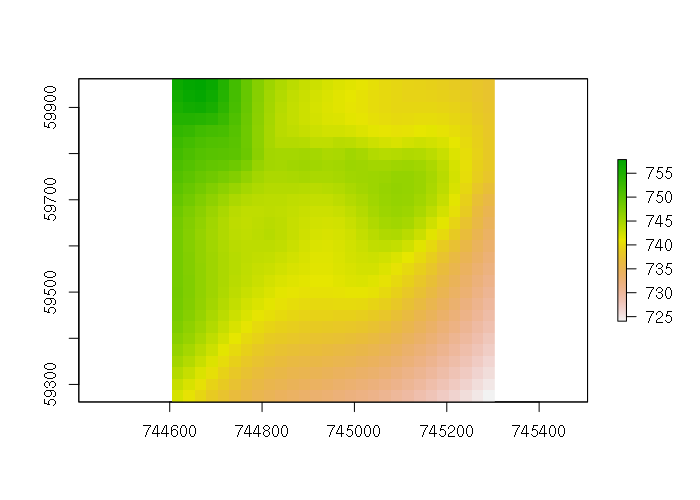Thin Plate Spline False Color Visualization

Creating a perspective visualization allows a richer view of the surface.

```persp(splined, scale=F, expand=15, shade=0.5, col='tan')
```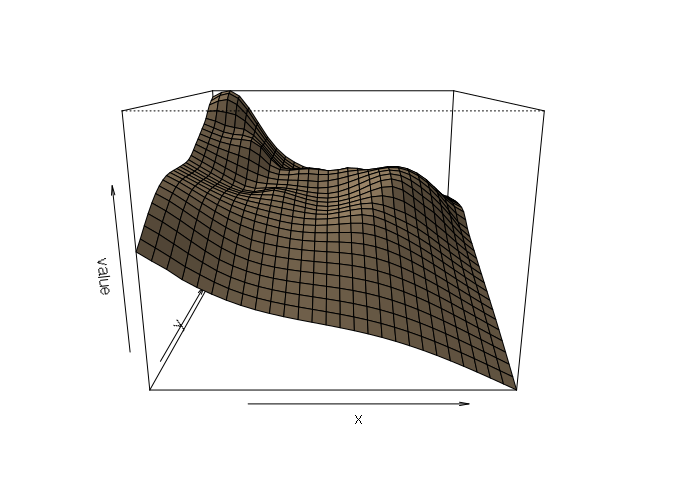Thin Plate Spline Perspective Visualization

Perspective plots can be false-colored to accentuate differences, although this is a bit cumbersome with persp(). Colors passed to persp() are the facets between points, not the points themselves, so the color matrix needs one fewer rows and one fewer columns. And the matrix needs to be transposed. And rows need to be reversed for some reason. These quirks explain the ugly formula on the first line of code below.

```colors = t(as.array(splined)[nrow(splined):2, 2:ncol(splined), 1])
colors = (colors - min(colors)) / diff(range(colors))
colors = rgb(colorRamp(c("#a6611a", "white", "#018571"))(colors), maxColorValue = 255)
persp(splined, col = colors, scale=F, expand=10)
```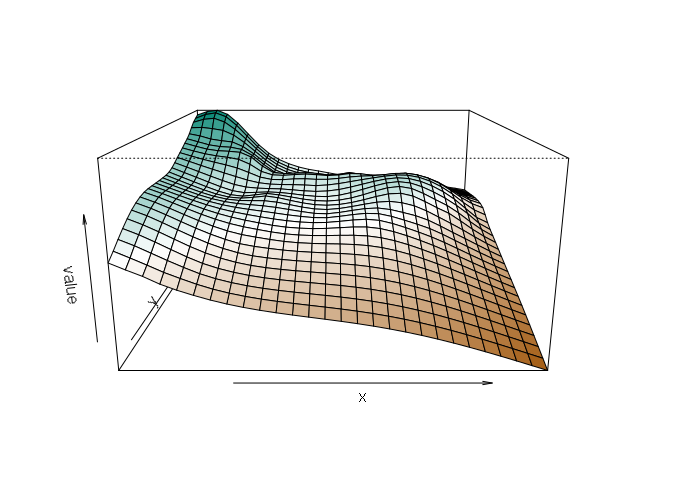Thin Plate Spline Perspective False-Color Visualization

## Inverse-Distance Weighting

Another simple model for interpolation is inverse-distance weighting, which interpolates points based on an average of all the sampled points, but which gives heigher weights to closer points so nearby points have more influence than more-distant points.

The gstat() function from the eponymous gstat library can extract the necessary values and distances from SpatialPointsDataFrame. The formula in this case tells gstat() to model the elevation attribute based on one times the inverse distance for each point.

```library(gstat)
model = gstat(formula = ele ~ 1, data = points)
idist = interpolate(template, model)
plot(idist)
```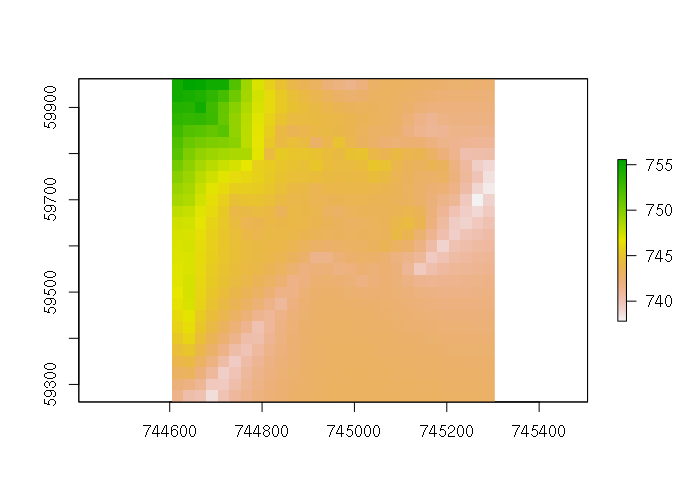Inverse Distance Weighted False-Color Visualization

This model brings out more fine-grained differences than the thin-plate spline, although in this case those differences are as much a result of GPS elevation inaccuracy as actual changes in elevation. This is made clearer in a perspective plot:

```persp(idist, scale=F, expand=15, shade=0.5, col='tan')
```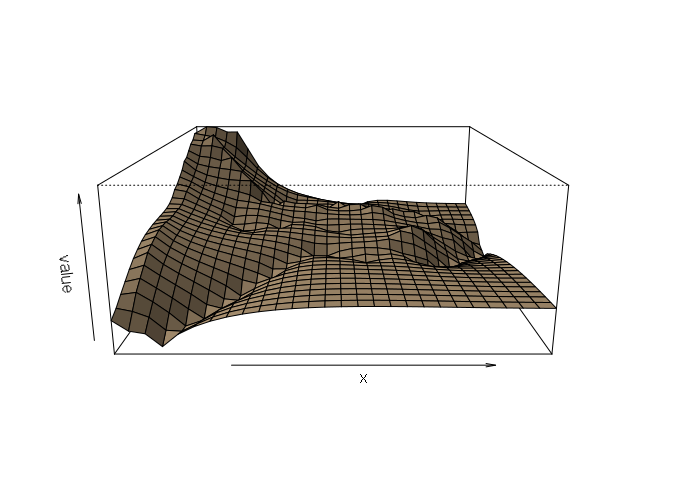Inverse Distance Weighted Perspective Visualization

## Ordinary Kriging

Perhaps the most common spatial interpolation technique is kriging, which was initially proposed by South African mining engineer Danie Gerhardus Krige in 1951 and further developed by later mathematicians. Krige is pronounced with a hard 'G' (rhymes with 'dig').

Kriging assumes that spatial variation in the phenomenon being estimated is statistically homogeneous throughout the surface. The same pattern of variation can be observed at all locations on the surface. Accordingly, ordinary kriging is not appropriate when dealing with surfaces known to have anomolous pits, spikes, or inconsistent changes, and inverse distance weighting might be the better choice. (ESRI 2008)

There are three types of kriging:

• Ordinary kriging involves fitting a simple curve to the variogram to model a single variable in terms of itself
• Universal kriging involves a model that considers a broad trend over the surface, a random, but spatially correlated component (like ordinary kriging and IDW), and random noise
• Co-Kriging involves a model built from kriging multiple variables

Ordinary kriging involves first creating a variogram which models how much two samples will differ from each other depending on the distance between those two samples.

Variograms can be created with the variogram() function from the gstat library. That variogram can then be viewed to determine the correct curve to fit to that model.

```library(gstat)
vario = variogram(ele ~ 1, data = points)
plot(vario)
```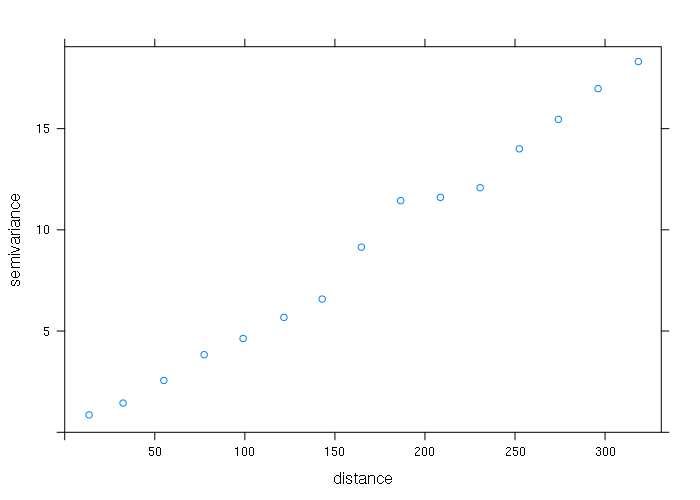Example Variogram

The safest model to start with is the Gaussian model. The vgm() function creates the model and the fit.variogram() adjusts the model parameters to fit the variogram.

```variogram_model = vgm(psill = max(vario\$gamma), model = 'Gau',
range = max(vario\$dist), nugget = min(vario\$gamma))

fitted_model = fit.variogram(vario, variogram_model)

plot(vario, model=fitted_model)
```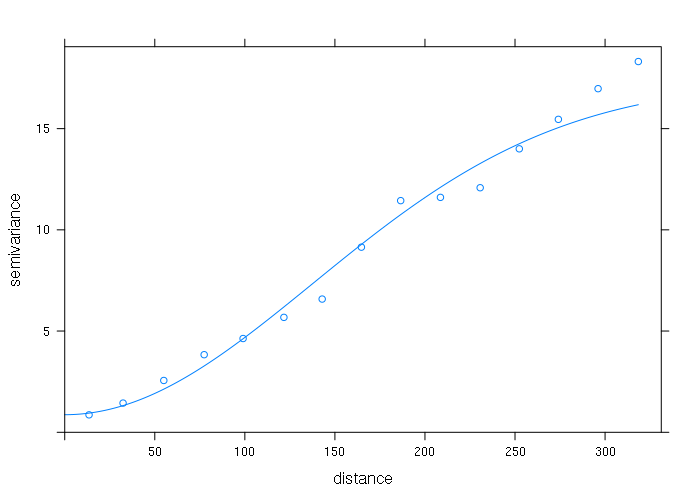Gaussian Model Fitted To a Variogram

That model can then be fed into gstat(), and the gstat model can be fed into the interpolate() function to create an interpolated surface.

```gstat_model = gstat(formula = ele ~ 1, data = points, model = fitted_model)
kriged = interpolate(template, gstat_model)
plot(kriged)
```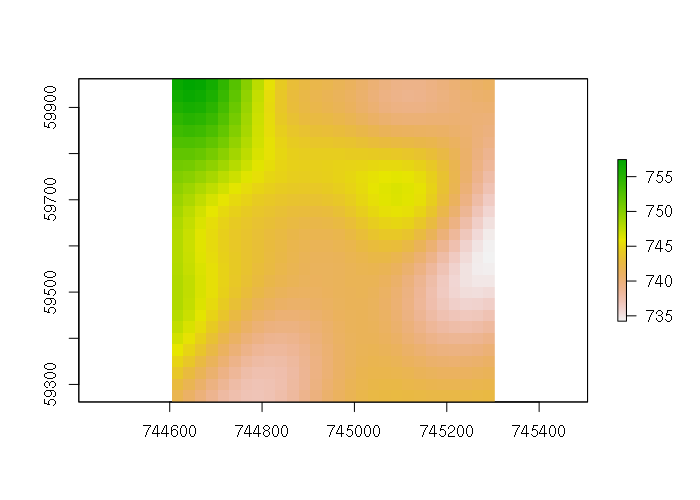Ordinary Kriging False-Color Visualization

The perspective plot shows the smoothing associated with the Gaussian curve:

```persp(kriged, scale=F, expand=15, shade=0.5, col='tan')
```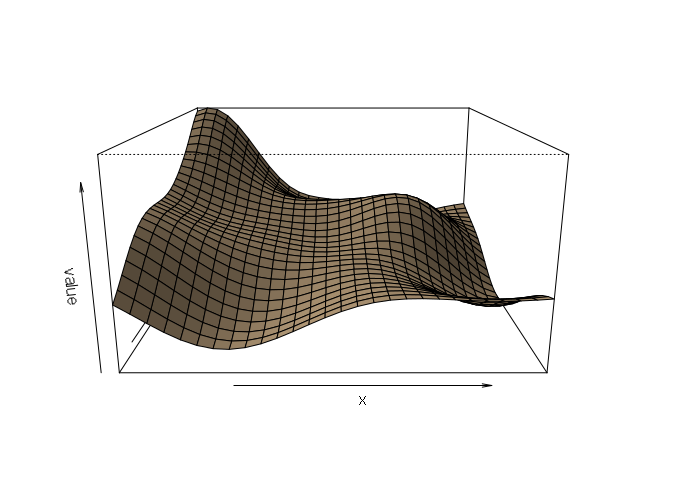Ordinary Kriging Perspective Visualization

There are a variety of different model curves and a variety of different parameters used to tweak those different models. However, the process can be a bit frustrating, and you might explore the autofitVariogram() function from the automap library if you are having issues getting fit.variogram() to fit your model.

For example, even though the variogram points form a fairly straight line, fit.variogram() issues a warning and subsequent interpolate() calls will fail.

```> linear_model = vgm(psill = max(vario\$gamma), model = 'Lin',
range = max(vario\$dist), nugget = min(vario\$gamma))
> linear_fit = fit.variogram(vario, linear_model)
Warning message:
In fit.variogram(vario, linear_model) : singular model in variogram fit
> gstat_model = gstat(formula = ele ~ 1, data = points, model = linear_fit)
> kriged = interpolate(template, gstat_model)
[using ordinary kriging]
There were 50 or more warnings (use warnings() to see the first 50)
```

## Comparing Methods

Using an actual DEM from the USGS, and reprojecting it to our area of analysis, we can see the actual elevation does not cleanly match the output of any of the interpolation methods. Ironically, the simplest of the models (thin-plate spline) probably gets closest, although the high error associated with the GPS data may also be a factor in poor model fit.

Nonetheless, this example dramatizes how different interpolation techniques can give different results with the same data, and that consideration of the underlying modeled phenomena is important in choosing an interpolation technique.

```actual = raster("imgn48w118_1.img")
actual = projectRaster(actual, template)
persp(actual, scale=F, expand=15, shade=0.5, col='tan')
```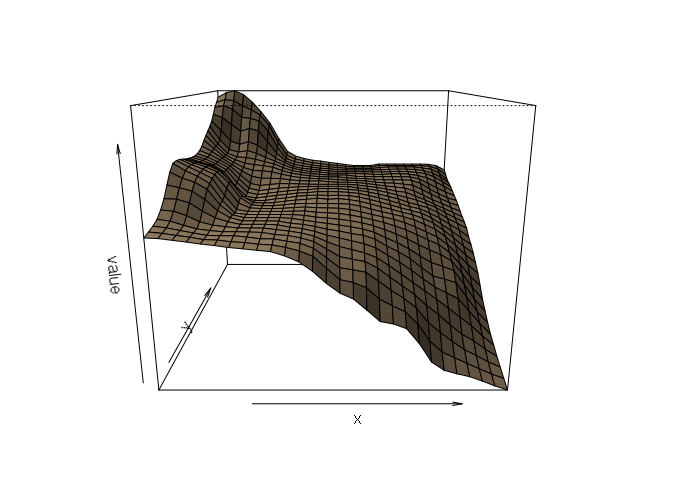Perspective Plot of USGS DEM DataThin Plate Spline Perspective VisualizationInverse Distance Weighted Perspective VisualizationOrdinary Kriging Perspective Visualization

## Kriging With Meuse Data

Many of the examples of Kriging on the web use the meuse data set that comes with the standard installation of R. This data set gives locations and topsoil heavy metal concentrations, along with a number of soil and landscape variables at the observation locations, collected in a flood plain of the river Meuse, near the village of Stein (NL). Heavy metal concentrations are from composite samples of an area of approximately 15 m x 15 m.

While these examples are often arcane in structure and difficult to relate to for people who are not soil scientists, this multivariable data set is especially useful to demonstrate universal kriging and co-kriging.

```library(sp)
data(meuse)

# Rijksdriehoek (RDH) (Netherlands topographical) map coordinates
netherlands = CRS("+init=epsg:28992 +towgs84=565.237,50.0087,465.658,-0.406857,0.350733,-1.87035,4.0812")
wgs84 = CRS("+proj=longlat +datum=WGS84 +no_defs")

points = SpatialPointsDataFrame(coords = meuse[,c('x','y')], data = meuse, proj4string = netherlands)
spplot(points, zcol='zinc')

library(OpenStreetMap)

keymap = openmap(upperLeft = c(51.0919, 5.5069), lowerRight = c(50.8523, 5.993), type="osm")
plot(keymap)
points(spTransform(points, osm()), pch=15, col='red', cex=0.5)
```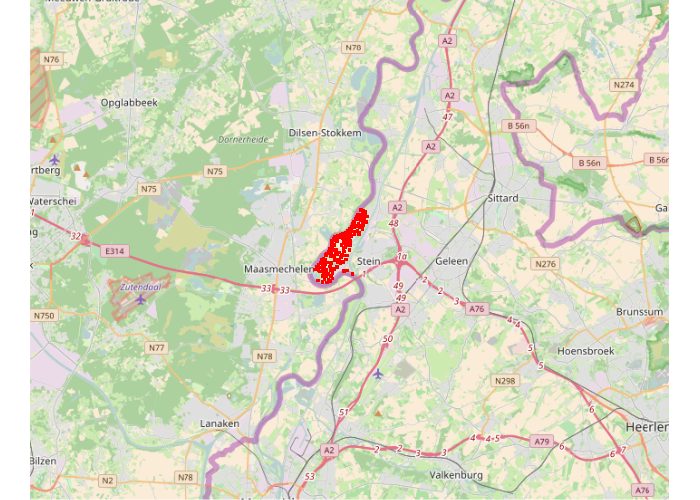Stein, Netherlands (Meuse River Valley)

### Surface Raster Template and OpenStreetMap Base Map

```pixelsize = 20
box = round(extent(points) / pixelsize) * pixelsize
template = raster(box, crs = netherlands, vals = 0,
nrows = (box@ymax - box@ymin) / pixelsize,
ncols = (box@xmax - box@xmin) / pixelsize)

library(OpenStreetMap)
outline = projectExtent(template, longlat())
basemap = openmap(c(ymax(outline), xmin(outline)), c(ymin(outline), xmax(outline)), type='stamen-toner')

```

### Ordinary Kriging

```vario = variogram(log(zinc) ~ 1, points)
fitted = fit.variogram(vario, vgm(1, "Sph", 300, 1))
plot(vario, model=fitted)
```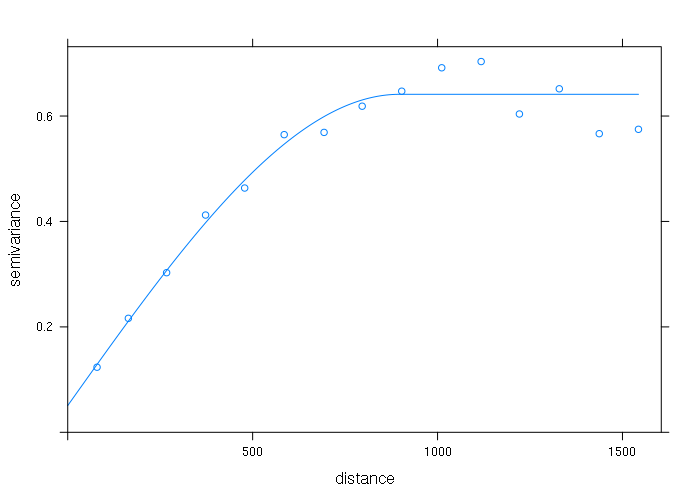Variogram of Meuse Zinc Measurement Autocorrelation
```gstat_model = gstat(formula = log(zinc) ~ 1, locations = points, model = fitted)
kriged = interpolate(template, gstat_model)

plot(basemap)
palette = colorRampPalette(c("#a6611ac0", "white", "#018571c0"), alpha=T)(256)
plot(projectRaster(kriged, projectExtent(kriged, osm())), col=palette, add=T)
```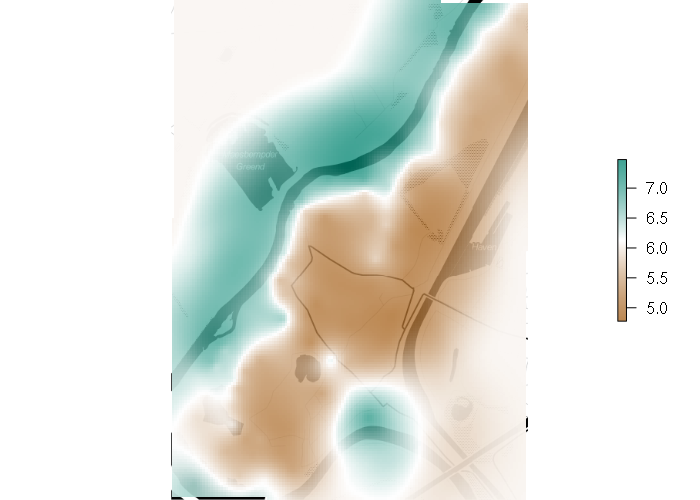Ordinary Kriging of Meuse Zinc Measurements

### Universal Kriging

Universal Kriging incorporates a model based on another spatial variable. In this case, zinc as a function of elevation.

```vario = variogram(log(zinc) ~ elev, points)
fitted = fit.variogram(vario, vgm(1, "Sph", 300, 1))
names(template) = 'elev'

gstat_model = gstat(formula = log(zinc) ~ elev, locations = points, model = fitted)
kriged = interpolate(template, gstat_model, xyOnly = F)

plot(basemap)
palette = colorRampPalette(c("#a6611ac0", "white", "#018571c0"), alpha=T)(256)
plot(projectRaster(kriged, projectExtent(kriged, osm())), col=palette, add=T)
```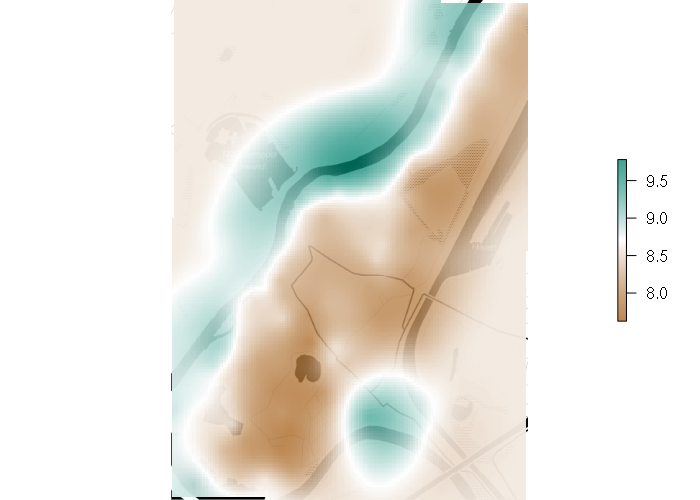Universal Kriging of Meuse Zinc Measurements Based on Elevation

### Co-Kriging

Co-Kriging involves performing kriging on multiple variables to build a model based on covariance with other variables. This model has the clearest definition of the group.

```model = gstat(formula = log(zinc) ~ 1, data = points)
model = gstat(g = model, formula = elev ~ 1, data = points)
model = gstat(g = model, formula = cadmium ~ 1, data = points)
model = gstat(g = model, formula = copper ~ 1, data = points)

covario = variogram(model)

cofitted = fit.lmc(covario, model, vgm(model = 'Sph', range = 1000))
cokriged = interpolate(template, cofitted)

plot(basemap)
palette = colorRampPalette(c("#a6611ac0", "white", "#018571c0"), alpha=T)(256)
plot(projectRaster(cokriged, projectExtent(cokriged, osm())), col=palette, add=T)
```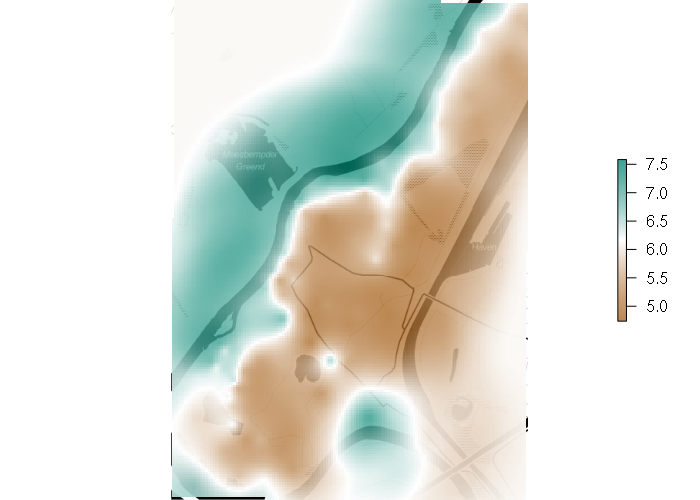Co-Kriging of Meuse Zinc Measurements